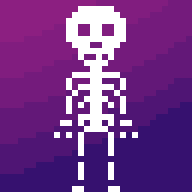Animate an 8 directional sprite in javascriptCodepen at bottom if you just want to look at the code without the walkthrough.

When animating a character for a game, you’ll usually be working with something called a “spritesheet”. Let’s take a look at the one we’re using for this tutorial:

So we have an 8x8 set of animation frames for a walking animation, at a pixel scale of 1. The columns each represent a different keyframe, and the rows represent different directions.

Let’s go through the pseudocode before we write any actual code. Our plan for animating this character is:

Now that we know what we’re trying to achieve, let’s go through the elements we’ll need.

Set up

1. Constants for the character’s dimensions and scale

2. An array of numbers corresponding to the number of keyframes.

3. Numbers corresponding to each direction assigned to constants representing those directions.

4. A constant to represent the delay between keyframes, and one to represent the character’s movement speed

5. A canvas element to draw onto.

6. Variables for the current direction, current keyframe, how many frames have passed on current keyframe, character coordinates, mouse coordinates, whether the mouse is present, and an image variable so we can preload the spritesheet to avoid loading it every time we change animation frames.

Writing functions

Since we want to allow the character to move in any direction, we can’t just change the x and y position at a fixed rate, or else the character will move faster on the diagonals than on horizontals and verticals. So, we need to do a little math. We’ll call the function we write here, called ‘angleMath’, whenever we want to set the deltas, angle, and distance vector:

Let’s add event listeners so we can detect the mouse. We’ll call ‘angleMath’ whenever the mouse moves inside the canvas:

In order to draw the character where we want, we’ll need to do a bit more math. Since we want the character to move in small increments and not travel the entire distance in an instant, we first reduce the delta X and Y distances by dividing by the vector distance from cursor to character. We then increment the x and y positions of the character by the deltas multiplied by the speed and scale variables. I added some basic collision code to prevent the character moving outside the borders, but you’ll want to use something different if you want to allow for more complex collision later on. Finally, we call angleMath again so that the character recalculates its angle to the target point as it moves. Without that, it will sometimes walk past the target point and continue in that direction forever.:

Now we need a function to actually draw the frames to the canvas. We’ll use the built-in function ‘drawImage’ to draw the frame onto the canvas’ context. The first argument is the spritesheet. The next two arguments represent which row and column we’re selecting the frame from. The next two dictate the size of the image to take from the source (the width and height of one frame), then the next two are the canvas dimensions, and finally the last two are the size that the image should be drawn onto the canvas.

Now we need a recursive function to first clear the canvas, then draw the correct frame, and repeat this process to create the animation.

First, clear the canvas to prepare it for drawing the next frame:

Next, choose the direction that the character should face based on the angle from the character to cursor:

Then check if the character should be moving or not. By setting a case of the hypotenuse of the coordinate deltas being less than or greater than a certain range, we can define the proximity to the cursor that defines when the character should stop moving:

If moving, run the animation, and if not, set the currentLoopIndex to the first keyframe which should be the character just standing. To run the animation, we increment the frameCount until it has passed the necessary amount of time, then reset the frameCount to zero and increment the currentLoopIndex to progress to the next keyframe:

Finally, draw that frame to the canvas and call the function within itself so that the animation will keep going. We use the built-in function, ‘requestAnimationFrame’, to accomplish this. The main purpose of using this function is to ensure that custom animations made in javascript run smoothly in the browser.

Now we’ve reached the end. Write a function to load the spritesheet and initialize the animation, then call that function: